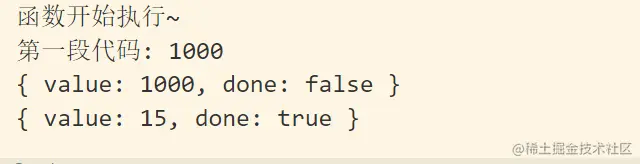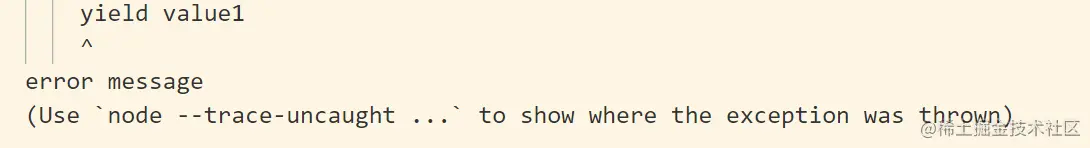# Iterator和Generator #

## Iterator #

### 什么是迭代器 #

• 迭代器协议定义了产生一系列值（无论是有限还是无限个）的标准方式。
• 在js中这个标准就是一个特定的next方法。

next方法有如下的要求：

• 一个无参数或者一个参数的函数，返回一个应当拥有以下两个属性的对象：
• done（boolean）
• 如果迭代器可以产生序列中的下一个值，则为 false。（这等价于没有指定 done 这个属性。）
• 如果迭代器已将序列迭代完毕，则为 true。这种情况下，value 是可选的，如果它依然存在，即为迭代结束之后的默认返回值。
• value
• 迭代器返回的任何 JavaScript 值。done 为 true 时可省略。但是一般将value设置为undefined。

### 实现一个迭代器 #

``````    function createArrayIterator(arr) {
let index = 0
return {
next: function() {
if (index < arr.length) {
return { done: false, value: arr[index++] }
} else {
return { done: true, value: undefined }
}
}
}
}

// 测试
const nums = [10, 22, 33, 12]
const numsIterator = createArrayIterator(nums)
console.log(numsIterator.next())
console.log(numsIterator.next())
console.log(numsIterator.next())
console.log(numsIterator.next())
console.log(numsIterator.next())

``````### 可迭代对象 #

• 它和迭代器是不同的概念。
• 当一个对象实现了可迭代协议（iterable protocol）时，它就是一个可迭代对象。
• 这个对象的要求是必须实现 @@iterator 方法，并且这个方法返回一个迭代器。在代码中我们使用 Symbol.iterator 访问该属性。

• 实现的时候注意this的指向。
``````    // 他需要具有[Symbol.iterator]方法，并且这个方法返回一个迭代器

const obj = {
names: ["zh", "llm"],
[Symbol.iterator]() {
let index = 0;
return {
next: () => {
if(index < this.names.length) {
return {
done: false, value: this.names[index++]
}
}else {
return {
done: true, value: undefined
}
}
}
}
}
}

``````

``````    for(let item of obj) {
console.log(item) // zh llm
}

``````

### 原生迭代器对象 #

String、Array、Map、Set、arguments对象、NodeList集合。

• 创建一些对象时：new Map([Iterable])、new WeakMap([iterable])、new Set([iterable])、new WeakSet([iterable])。
• 一些方法的调用：Promise.all(iterable)、Promise.race(iterable)、Array.from(iterable)。

### 自定义类的迭代 #

``````    class Classroom {
this.name = name
this.students = students
}

entry(newStudent) {
this.students.push(newStudent)
}

[Symbol.iterator]() {
let index = 0
return {
next: () => {
if (index < this.students.length) {
return { done: false, value: this.students[index++] }
} else {
return { done: true, value: undefined }
}
},
return: () => {
console.log("迭代器提前终止了~")
return { done: true, value: undefined }
}
}
}
}

``````

• 比如遍历的过程中通过break、continue、return、throw中断了循环操作
• 比如在解构的时候，没有解构所有的值

``````    for (const stu of classroom) {
// 通过break中断
if (stu === "zh") break
}

``````

## Generator #

### 什么是生成器 #

• 首先，生成器函数需要在function的后面加一个符号：`*`
• 其次，生成器函数可以通过yield关键字来控制函数的执行流程。
• 最后，生成器函数的返回值是一个Generator（生成器）。

``````    function* fon 我们发现上面的生成器函数foo的执行体压根没有执行，它只是返回了一个生成器对象。

p那么我们如何可以让它执行函数中的东西呢？调用next即可；

p我们之前学习迭代器时，知道迭代器的next是会有返回值的；

p但是我们很多时候不希望next返回的是一个undefined，这个时候我们可以通过yield来返回结果；o() {
console.log("函数开始执行~")

const value1 = 100
console.log("第一段代码:", value1)
yield

const value2 = 200
console.log("第二段代码:", value2)
yield

const value3 = 300
console.log("第三段代码:", value3)
yield

console.log("函数执行结束~")
}

// 调用生成器函数时, 会给我们返回一个生成器对象
const generator = foo()

``````

### 生成器函数执行 #

``````    function* foo() {
console.log("函数开始执行~")

const value1 = 100
console.log("第一段代码:", value1)
yield value1

const value2 = 200
console.log("第二段代码:", value2)
yield value2

const value3 = 300
console.log("第三段代码:", value3)
yield value3

console.log("函数执行结束~")
return "123"
}

``````

### 生成器传递参数 – next函数 #

``````    // 伪代码
接收参数 = yeild
next(参数)

``````

### 生成器提前结束 – return函数 #

return传值后这个生成器函数就会结束，之后调用next不会继续生成值了。其实他就是直接return函数。并且把传递的参数作为return的值。

``````function* foo(num) {
console.log("函数开始执行~")

const value1 = 100 * num
console.log("第一段代码:", value1)
const n = yield value1

// 调用return方法后相当于
// return n;

const value2 = 200 * n
console.log("第二段代码:", value2)
const count = yield value2

const value3 = 300 * count
console.log("第三段代码:", value3)
yield value3

console.log("函数执行结束~")
return "123"
}

const generator = foo(10)

console.log(generator.next())

// 第二段代码的执行, 使用了return
// 那么就意味着相当于在第一段代码的后面加上return, 就会提前终端生成器函数代码继续执行
console.log(generator.return(15))

``````由上面的执行结果来看，调用return方法后，函数直接被终止。然后将传入的值作为迭代器对象的value，并且将done改为true。

### 生成器抛出异常 – throw函数 #

• 抛出异常后我们可以在生成器函数中捕获异常。
• 并且抛出的异常是上一次yeild的代码片段。
• 如果使用try...catch捕获到异常，那么我们可以向throw传递参数作为catch的参数。并且后续代码还可以继续调用next执行。
• 如果不捕获异常，后续代码将不能继续调用next执行。
``````    function* foo() {
console.log("代码开始执行~")

const value1 = 100
//try {
yield value1
// } catch (error) {
//  console.log("捕获到异常情况:", error)
//}

console.log("第二段代码继续执行")
const value2 = 200
yield value2

console.log("代码执行结束~")
}

const generator = foo()

const result = generator.next()
generator.throw("error message")
generator.next()

``````### 生成器替代迭代器 #

``````    function createArrayIterator(arr) {
let index = 0
return {
next: function() {
if (index < arr.length) {
return { done: false, value: arr[index++] }
} else {
return { done: true, value: undefined }
}
}
}
}

``````

• 方法一： 直接取出传入的数据，然后yield返回。
• 方法二: 其实和方法一一样。
• 方法三：使用`yield*`来生产一个可迭代对象。这个时候相当于是一种yield的语法糖，只不过会依次迭代这个可迭代对象，每次迭代其中的一个值。
``````// 1.生成器来替代迭代器
function* createArrayIterator(arr) {

// 3.第三种写法 yield*
// yield* arr

// 2.第二种写法
// for (const item of arr) {
//   yield item
// }

// 1.第一种写法
for(let i = 0; i < arr.length; i++) {
yield arr[i];
}
}

const names = ["zh", "llm", "zhllm"]
const namesIterator = createArrayIterator(names)

console.log(namesIterator.next())
console.log(namesIterator.next())
console.log(namesIterator.next())
console.log(namesIterator.next())

``````

### 自定义类迭代 – 生成器实现 #

``````    class Classroom {
this.name = name
this.students = students
}

// [Symbol.iterator] = function*() {
//   yield* this.students
// }

*[Symbol.iterator]() {
yield* this.students
}
}

``````

### 异步处理方案 #

``````    function requestData(url) {
return new Promise((resolve, reject) => {
// 模拟网络请求
setTimeout(() => {
// 拿到请求的结果
resolve(url)
}, 2000);
})
}

``````
• 方案一： 多次调用requestData函数
``````    // 1.第一种方案: 多次回调
// 回调地狱
requestData("zh").then(res => {
requestData(res + "aaa").then(res => {
requestData(res + "bbb").then(res => {
console.log(res) //等待六秒，打印结果 zhaaabbb
})
})
})

``````
• 方案二： 多次调用then函数，因为then的状态是有返回的Promise决定的。
``````    // 2.第二种方案: Promise中then的返回值来解决
requestData("zh").then(res => {
return requestData(res + "aaa")
}).then(res => {
return requestData(res + "bbb")
}).then(res => {
console.log(res) //等待六秒，打印结果 zhaaabbb
})

``````
• 方案三：通过Promise + generator实现。手动调用generator函数来自执行迭代器对象。
``````    // 3.第三种方案: Promise + generator实现
function* getData() {
const res1 = yield requestData("zh")
const res2 = yield requestData(res1 + "aaa")
const res3 = yield requestData(res2 + "bbb")
const res4 = yield requestData(res3 + "ccc")
}

// 1> 手动执行生成器函数
const generator = getData()
generator.next().value.then(res => {
console.log("res----1",res) // zh
generator.next(res).value.then(res => {
console.log("res----2",res) // zhaaa
generator.next(res).value.then(res => {
console.log("res----3",res) // zhaaabbb
generator.next(res).value.then(res => {
console.log("res----4",res) // zhaaabbbccc
})
})
})
})

``````
• 由于上面代码是手动调用，封装一个自动调用迭代器对象的函数。
``````    function* getData() {
const res1 = yield requestData("zh")
const res2 = yield requestData(res1 + "aaa")
const res3 = yield requestData(res2 + "bbb")
const res4 = yield requestData(res3 + "ccc") // 这里返回的依旧是done: false
return res4 // 这里最后返回 {done: true, value: res4}
}

// 封装了一个自动执行的函数
function execGenerator(genFn) {
const generator = genFn()
function exec(res) {
const result = generator.next(res)
if (result.done) {
console.log(result.value) // zhaaabbbccc
return result.value
}
result.value.then(res => {
exec(res)
})
}
exec()
}

execGenerator(getData)

``````
• 第三方包co自动执行
``````    function* getData() {
const res1 = yield requestData("zh")
const res2 = yield requestData(res1 + "aaa")
const res3 = yield requestData(res2 + "bbb")
const res4 = yield requestData(res3 + "ccc")
}
const co = require('co')
co(getData)

``````
• 使用es8推出的async, await。
``````    async function getData() {
const res1 = await requestData("zh")
const res2 = await requestData(res1 + "aaa")
const res3 = await requestData(res2 + "bbb")
const res4 = await requestData(res3 + "ccc")
console.log(res4)
}

getData()

``````